Next: Linearized Supersonic Flow Up: Two-Dimensional Compressible Inviscid Flow Previous: Supersonic Flow Past a

# Linearized Subsonic Flow

The aim of this section is to modify the two-dimensional, incompressible, subsonic aerodynamic theory discussed in Chapter 9 so as to take the finite compressibility of air into account.

Consider compressible, subsonic flow over a thin airfoil at a small angle of attack. Such a situation can be analyzed using the small-perturbation theory introduced in Section 15.12. As before, the unperturbed flow is of uniform speed, directed parallel to the-axis, and the associated Mach number is. The perturbed flow, due to the presence of the airfoil, is governed by Equation (15.127), which can be written in the elliptic form (see Section 15.13)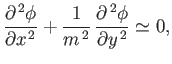(15.164)

where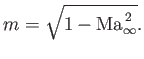(15.165)

Equation (15.164) can be transformed into a familiar incompressible form by introducing a transformed coordinate system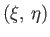, such that(15.166)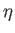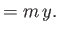(15.167)

In this transformed space, we define a transformed perturbed velocity potential,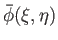, such that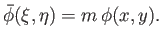(15.168)

Now,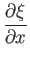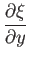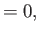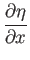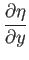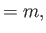(15.169)

so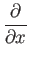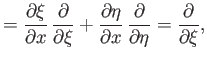(15.170)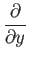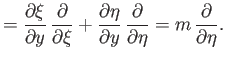(15.171)

It follows that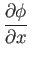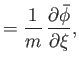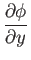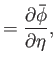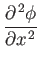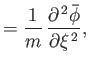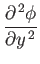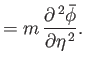(15.172)

Thus, Equation (15.164) transforms to give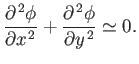(15.173)

However, this is simply Laplace's equation, which governs incompressible flow. (See Section 4.15.) Hence,represents an incompressible flow inspace that is related to a compressible flow, represented by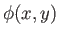, in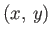space.

Suppose that the surface of the airfoil is given by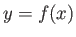inspace, and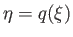inspace. According to Equation (15.129), the boundary condition on the flow at the airfoil surface is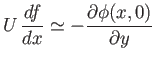(15.174)

inspace. The analogous boundary condition inspace is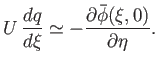(15.175)

However,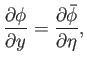(15.176)

which implies that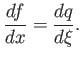(15.177)

In other words, the shape of the airfoil in (,) space is the same as that in (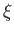,) space. Hence, the coordinate transformation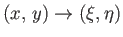transforms compressible flow over an airfoil inspace to incompressible flow over the same airfoil inspace.

According to Equation (15.128), the pressure coefficient inspace is written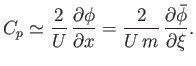(15.178)

The corresponding incompressible pressure coefficient inspace is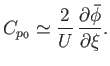(15.179)

A comparison of the previous two equations reveals that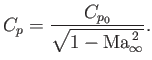(15.180)

This result is known as the Prandtl-Glauert rule: it is a similarity rule that relates incompressible flow over a given two-dimensional airfoil to subsonic compressible flow over the same airfoil. Given that the coefficient of lift is directly related to the pressure coefficient (see Section 15.9), we can also deduce that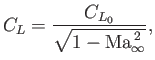(15.181)

where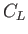is the coefficient of lift for compressible flow, and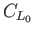the corresponding coefficient for incompressible flow. It is clear that compressibility has the effect of increasing the lift on a given airfoil.

Equation (15.181) indicates that the coefficient of lift goes to infinity asapproaches unity, which is an impossible result. The quandary is resolved by recalling that linearized theory breaks down in the transonic regime (where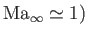. In fact, the Prandlt-Glauert rule is only accurate up to Mach numbers of approximately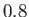.

The effect of compressibility on subsonic flow-fields can be seen by noting that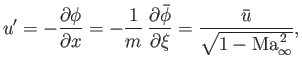(15.182)

where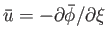is the incompressible velocity perturbation parallel to the unperturbed flow. It can be seen that asincreases, the parallel velocity perturbation,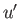, also increases relative to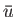. In other words, compressibility strengthens the disturbance of the flow introduced by the airfoil.

In conventional inviscid, incompressible aerodynamic theory, a two-dimensional airfoil experiences zero aerodynamic drag (assuming that there is no separation of the boundary layer). (See Chapters 8 and 9.) This is due to the fact that, in the absence of friction, the pressure distributions over the forward and rearward portions of the airfoil exactly cancel in the direction of the unperturbed flow. Note that the compressible pressure coefficient,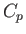, only differs from the incompressible pressure coefficient,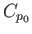, by a constant scale-factor. Thus, if the distribution ofover the surface of the airfoil results in zero drag then the distribution ofwill also result in zero drag. In other words, in subsonic flow, compressibility does not give rise to additional aerodynamic drag.Next: Linearized Supersonic Flow Up: Two-Dimensional Compressible Inviscid Flow Previous: Supersonic Flow Past a
Richard Fitzpatrick 2016-03-31